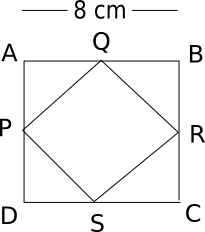Question 40

# A square is drawn by joining the midpoints of the sides of a given square. A third square is drawn inside the second square in the same way and this process is continued indefinitely. If a side of the first square is 8 cm, the sum of the areas of all the squares such formed (in sq.cm.)is

SolutionSide of first square = 8cm.

Side of second square made by joining mid-points of first square = $$\frac{8}{\sqrt2}$$

Similarly side of third square =  $$\frac{8}{\sqrt2\times\sqrt2}$$ and so on.

Now summation of areas will be $$8^2+(\frac{8}{\sqrt2})^2+(\frac{8}{\sqrt2\times\sqrt2})^2$$ .........

or $$8^2 ( 1+\frac{1}{2}+\frac{1}{4}+\frac{1}{8}.....)$$

or $$64 \times (\frac{1}{1-\frac{1}{2}})$$ (As we know sum of an infinite G.P. is $$\frac{a}{1-r}$$ where a is first term and r is common ratio)

or 128 sq. cm.